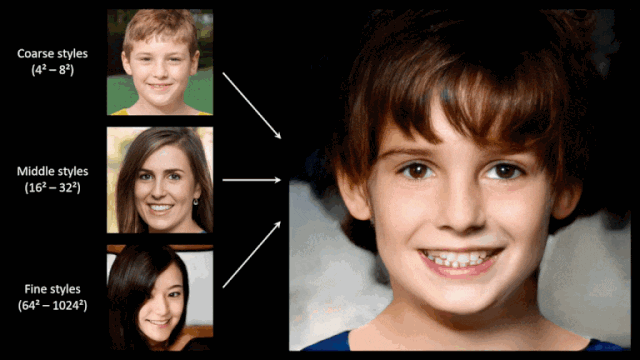# Generative Adversarial Network to create meaningful images from noises

Original article was published on Deep Learning on Medium# Generative Adversarial Network to create meaningful images from noises

In this article, we will learn how to build a GAN from scratch using Convolution layers. For demonstration and quick work out, we will be using the Fashion MNIST dataset. And after you learn it, similarly you can do for faces. Before going into details I want you to think, Can we use Deep learning to create something from nothing? To answer this question we are going to implement a GAN and see the results with our own eyes.

GAN is a unification of two Neural networks: Generator and Discriminator. The generator, generates new data instances, while the other, the discriminator, evaluates them for authenticity; i.e. the discriminator decides whether each instance of data that it reviews belongs to the actual training dataset or not. Now, let us implement and see it ourself.

1. Tensorflow ( I tested using 1.12)
2. Keras
3. Pandas
4. OpenCV

You can now start by opening a Jupyter-notebook

`# Import all the librariesimport kerasimport pandas as pdimport numpy as npimport matplotlib.pyplot as pltfrom keras.layers import Dense, Dropout, Input, Conv2D, Flatten, BatchNormalization, Reshapefrom keras.models import Model,Sequentialfrom keras.datasets import fashion_mnistfrom tqdm import tqdmfrom keras.layers.advanced_activations import LeakyReLUfrom keras.optimizers import adam`

`(x_train,y_train), (x_test, y_test) = fashion_mnist.load_data()#We next normalize the imagesx_train = (x_train.astype(np.float32) - 127.5)/127.5`

Now we have the training data available, which contains 60K images with shape (28x28x1). So, we need to create a Generator which accepts images of that particular shape.

`def create_generator(): generator=Sequential() generator.add(Conv2D(64, (3,3), strides =(3,3), padding ='same', input_shape = [28,28,1])) generator.add(BatchNormalization()) generator.add(LeakyReLU(0.2)) generator.add(Conv2D(32, (3,3), strides =(3,3), padding ='same')) generator.add(BatchNormalization()) generator.add(LeakyReLU(0.2)) generator.add(Flatten()) generator.add(Dense(units=512)) generator.add(LeakyReLU(0.2)) generator.add(Dense(units=1024)) generator.add(LeakyReLU(0.2)) generator.add(Dense(units=28*28, activation='tanh')) #generator.add(Reshape((28,28))) generator.compile(loss='binary_crossentropy', optimizer=adam_optimizer()) return generatorg=create_generator()g.summary()#Summary of the GeneratorLayer (type) Output Shape Param # ================================================================= conv2d_3 (Conv2D) (None, 10, 10, 64) 640 _________________________________________________________________ batch_normalization_3 (Batch (None, 10, 10, 64) 256 _________________________________________________________________ leaky_re_lu_13 (LeakyReLU) (None, 10, 10, 64) 0 _________________________________________________________________ conv2d_4 (Conv2D) (None, 4, 4, 32) 18464 _________________________________________________________________ batch_normalization_4 (Batch (None, 4, 4, 32) 128 _________________________________________________________________ leaky_re_lu_14 (LeakyReLU) (None, 4, 4, 32) 0 _________________________________________________________________ flatten_3 (Flatten) (None, 512) 0 _________________________________________________________________ dense_15 (Dense) (None, 512) 262656 _________________________________________________________________ leaky_re_lu_15 (LeakyReLU) (None, 512) 0 _________________________________________________________________ dense_16 (Dense) (None, 1024) 525312 _________________________________________________________________ leaky_re_lu_16 (LeakyReLU) (None, 1024) 0 _________________________________________________________________ dense_17 (Dense) (None, 784) 803600 ================================================================= Total params: 1,611,056 Trainable params: 1,610,864 Non-trainable params: 192`

We have created a Generator which consists of two Convolutional layers followed by two dense layers. The final dense layer has the shape (28×28) so that we can reconstruct it back to images as in the dataset.

Next, we create the discriminator which is going to determine if the images created by the Generator are fake or Real. If the discriminator predicts those images to be fake, then the generator modifies its weight until it can cheat the discriminator. This is how the Generator is going to learn to create images out of noises.

`def create_discriminator(): discriminator=Sequential() discriminator.add(Dense(units=512,input_dim=28*28)) #discriminator.add(Conv2D(64, (3,3), strides =(1,1), padding ='same', input_shape = [28,28,1])) discriminator.add(LeakyReLU(0.2)) discriminator.add(Dropout(0.3)) #discriminator.add(Flatten()) discriminator.add(Dense(units=512)) discriminator.add(LeakyReLU(0.2)) discriminator.add(Dropout(0.3)) discriminator.add(Dense(units=256)) discriminator.add(LeakyReLU(0.2)) discriminator.add(Dense(units=1, activation='sigmoid')) discriminator.compile(loss='binary_crossentropy', optimizer=adam_optimizer()) return discriminatord =create_discriminator()d.summary()`

We have created both the Generator and the Discriminator. Next, we unify both of them to create the GAN. In the code below, we unify the two modules and we train them.

`def create_gan(discriminator, generator): discriminator.trainable=False img_shape=(28, 28, 1) gan_input = Input(img_shape) x = generator(gan_input) gan_output= discriminator(x) gan= Model(inputs=gan_input, outputs=gan_output) gan.compile(loss='binary_crossentropy', optimizer=adam_optimizer()) return gan`

Now let us train the GAN

`# We complete the GAN by unifying Generator with the Discriminatorgenerator= create_generator()discriminator= create_discriminator()gan = create_gan(discriminator, generator)batch_size = 128Epochs = 300for epcs in range(1, Epochs ): print("Epoch Number %d" %epcs) for _ in tqdm(range(batch_size)): #generate random noise of shape 28*28 to initialize the generator noise= np.random.normal(0,1, [batch_size, 28,28,1]) # Now predict the noise by feeding it to generator generated_images = generator.predict(noise) # Take real images from the Fashion MNIST and reshape it for the discriminator image_batch =x_train[np.random.randint(low=0,high=x_train.shape,size=128)] # The discriminator accepts vector of dimension 784, and hence we reshape the images image_batch = image_batch.reshape(-1,28*28) #We concate both real and fake images to train the discriminator X= np.concatenate([image_batch, generated_images]) # We Next assign label to X y_discriminator=np.zeros(2*batch_size) # First images are true, so we label them 1 y_discriminator[:batch_size]=1 #Pre train discriminator on fake and real data before starting the gan.  discriminator.trainable=True discriminator.train_on_batch(X, y_discriminator) # We trick the noises to be real, so that the GAN eventually learns noise= np.random.normal(0,1, [batch_size, 28,28,1]) y_label = np.ones(batch_size) # While traininig the GAN, we dont want to alter the weights of the discriminator discriminator.trainable=False # Let us train the GAN  gan.train_on_batch(noise, y_label)**************OUTPUTS*************Epoch 1100%|██████████| 128/128 [00:03<00:00, 37.96it/s] 3%|▎ | 4/128 [00:00<00:03, 38.77it/s]Epoch 2100%|██████████| 128/128 [00:03<00:00, 37.25it/s] 3%|▎ | 4/128 [00:00<00:03, 38.01it/s]`

After the training is over you can predict the Generator based on the noise.

`# feed the noises to the generator and predict themgenerated_images = generator.predict(noise)#we are going to see the first image i.e at index 0x =generated_images.reshape(28,28)# Let us plot the imageplt.imshow(x, cmap ="Greys")`